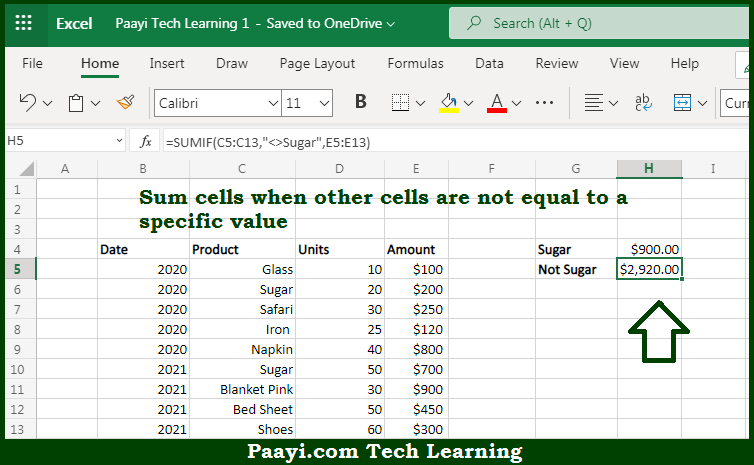# Learn How to SUM If Cells Are Not Equal To in Microsoft Excel

Written by | 0 Comments | 572 Views

In this article, you will learn how to SUM various things in Microsoft Excel using a single/combination(s) of functions. You will also know how to SUM If Cells Are Not Equal To and see the generic formula.

SUM If Cells Are Not Equal To in Microsoft Excel

The main purpose of this formula is to sum cells when other cells are not equal to a specific value. Here we will learn how to sum if cells are equal to in the workbook in Microsoft Excel. That implies, with the help of a formula based on the SUMIFS function you can able to sum cells when other cells are not equal to a specific value. So, with the help of this formula, you can able to sum if cells are equal to in the workbook in Microsoft Excel.

General Formula to SUM If Cells Not Are Equal To

=SUMIF(range,"<>value",sum_range)

The Explanation for the SUM If Cells Are Not Equal ToSo we know that with the help of the given formula above you can able to sum cells when other cells are not equal to a specific value. Here we will learn how to sum if cells are equal to in the workbook in Microsoft Excel. As we know that the SUMIF function supports all of the standard operators provided in Microsoft Excel. That includes not-equal-to, which is input as <>. When you use an operator in the criteria for a function like SUMIF, you need to enclose it in double quotes (" "). So, with the help of this formula, you can able to sum if cells are equal to in the workbook in Microsoft Excel.